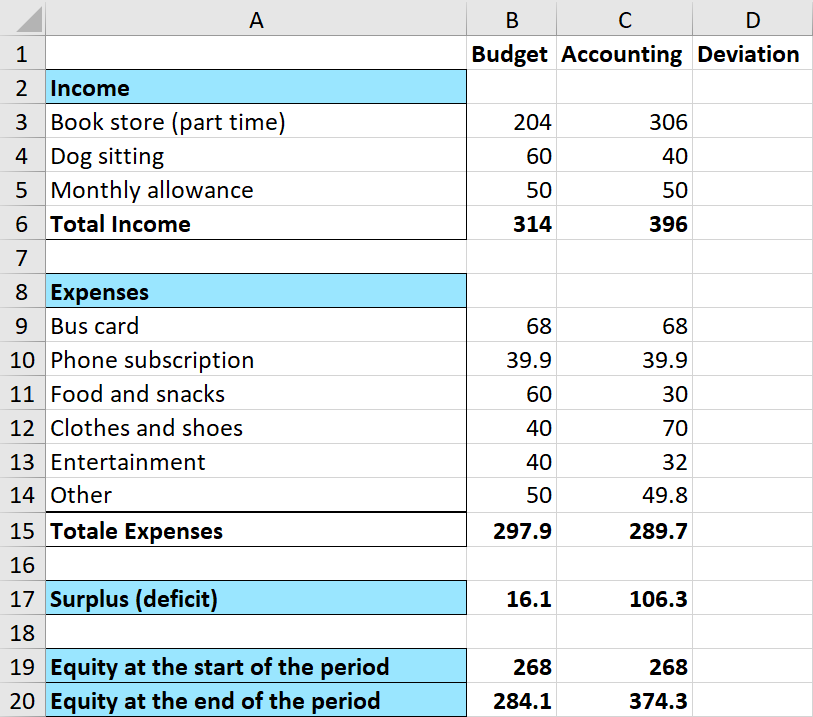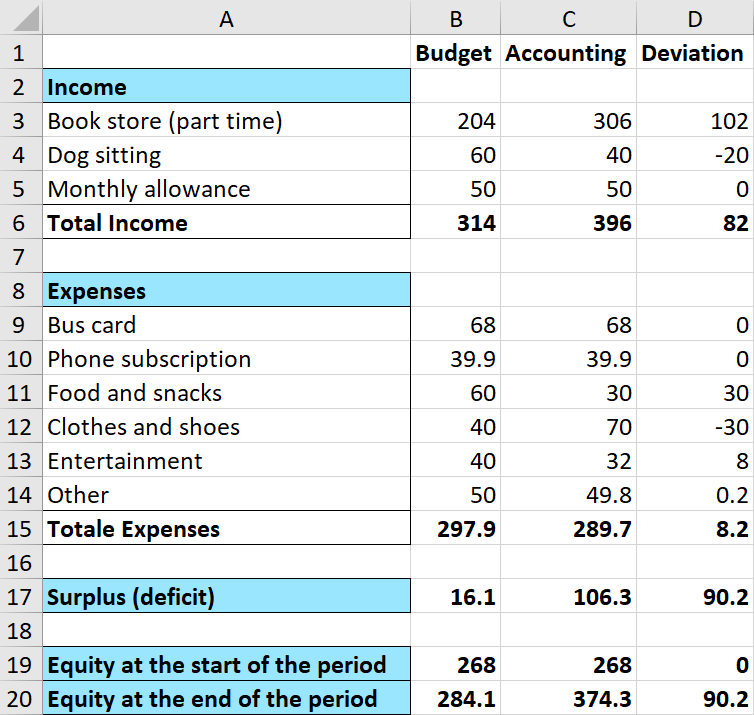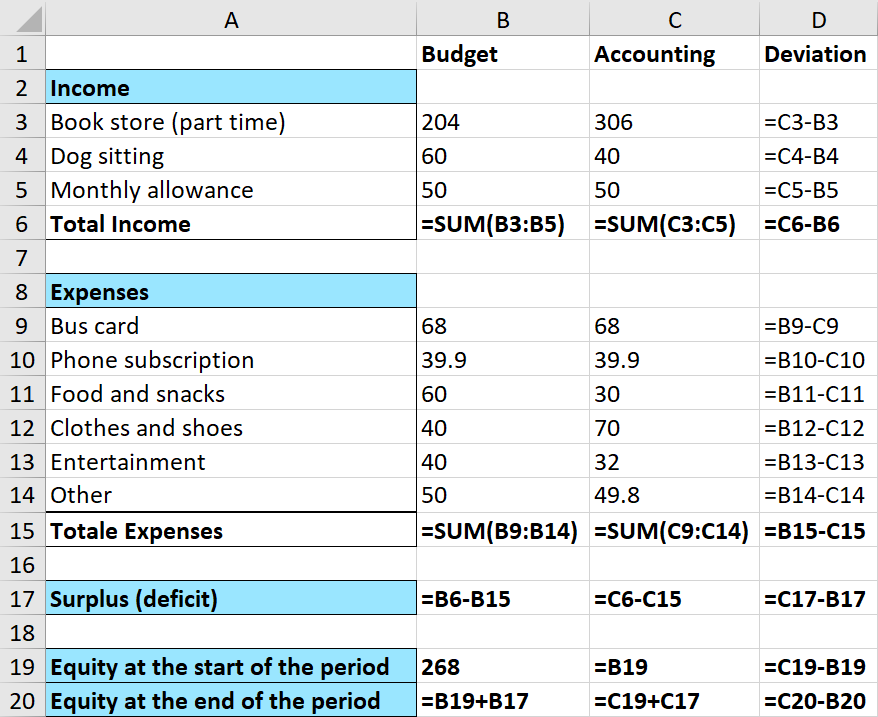# Comparing Budget and Accounting in Excel

It’s very useful to compare a budget to an accounting, and you will now learn how to do this efficiently in `Excel`.

`Excel` Instruction

### ComparingaBudgettoanAccounting

1.
Create a budget and an accounting spreadsheet.
2.
Create the “skeleton” of the table.
3.
Enter the numbers from your budget, accounting and the starting equity—the amount you had in reserve at the beginning.
4.
Write the formulas for “deviation” in each category. Sum these deviations so you can compare the budgeted surplus/ deficit with the actual surplus/ deficit. A surplus will be added to the existing equity, while a deficit will reduce the equity.
5.
Finally, you should consider making changes to your budgeting process based on the information in the table. There can, for example, be a big difference between certain items in the budget, and what their actual values are in the accounting.

Example 1

Erica Clementine wants to compare her estimated income and expenses (budget) with her actual income and expenses (accounting) in a month. She has \$$\text{}268\text{}$ in equity at the beginning of the month. She needs your help to set up an overview of her personal economic situation.

1.
The first thing you need to do is make a budget and an accounting of Erica Clementine’s situation.
2.
Then you make a “skeleton table” in `Excel`. Notice that the setup is slightly different from the budget and accounting setup.
3.
Input the numbers from the budget and accounting, and the starting equity, into the skeleton table. Your spreadsheet should look like this:4.
Now enter the formulas in the column for “deviation”. This is the deviation between the budget and the accounting for each category.

Note! For income you subtract the budget number from the accounting number, while for expenses you subtract the accounting number from the budget number. This is because you want a positive deviation to be good for the person or the company the table is for. It’s a good thing if the actual income is greater than the assumed income. When it comes to expenses, you want the actual expenses to be less than the assumed expenses.

The budgeted profit (deficit) and the actual profit (deficit) is added to the beginning equity to find the equity in the end of the period. Below you’ll see the numbers and formulas of the table.5.
Finally, you’ll take a look at the table to see if there’s any categories with a significant deviation. An interesting observation is that Erica Clementine worked more in the bookstore than what was budgeted, which may lead to her not earning as much as planned as a dog sitter. In total, her income was higher than planned.

If you look at the expenses you can see that the total expenses are approximately as planned, and while her food and snacks expenses increased, this was made up for with a decrease in clothes and shoes expenses.

Erica Clementine budgeted for a profit of \$$16.10$, but actually had a profit of \$$106.30$. The main reason for this deviation of the profit is due to the increased income from the bookstore. She increased her equity this month from \$$268$ to \$$374.30$.